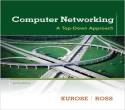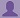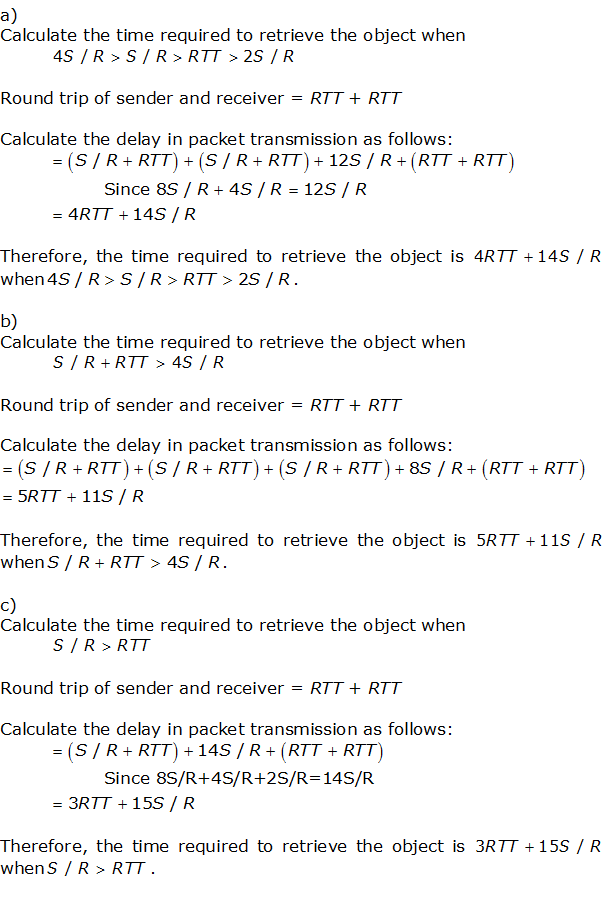SHARE
HELP

To start with, Sr2Jr’s first step is to reduce the expenses related to education. To achieve this goal Sr2Jr organized the textbook’s question and answers. Sr2Jr is community based and need your support to fill the question and answers. The question and answers posted will be available free of cost to all.# Computer Networking : A Top-down Approach

Authors:
James F. Kurose, Keith W. Ross
Exercise:
Problems
Chapter:
Transport Layer
Edition:
6
ISBN:
9780132856201
Question:
56
 Previous Next

###Question

In this problem, we consider the delay introduced by the TCP slow-start phase. Consider a client and a Web server directly connected by one link of rate R. Suppose the client wants to retrieve an object whose size is exactly equal to 15 S, where S is the maximum segment size (MSS). Denote the round-trip time between client and server as RTT (assumed to be constant).

Ignoring protocol headers, determine the time to retrieve the object (including TCP connection establishment) when

a. 4 S/R > S/R + RTT > 2S/R

b. S/R + RTT > 4 S/R

c. S/R > RTT.

###Answer

Consider the following data:

The transmission rate of Client and Web = R.

Maximum Segment Size (MSS) = S

Round Trip Time (RTT) between client and server is constant.### Discussions

Post the discussion to improve the above solution.